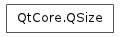QSizeF

QMargins

# QSize¶## Detailed Description¶

The PySide.QtCore.QSize class defines the size of a two-dimensional object using integer point precision.

A size is specified by a PySide.QtCore.QSize.width() and a PySide.QtCore.QSize.height() . It can be set in the constructor and changed using the PySide.QtCore.QSize.setWidth() , PySide.QtCore.QSize.setHeight() , or PySide.QtCore.QSize.scale() functions, or using arithmetic operators. A size can also be manipulated directly by retrieving references to the width and height using the PySide.QtCore.QSize.rwidth() and PySide.QtCore.QSize.rheight() functions. Finally, the width and height can be swapped using the PySide.QtCore.QSize.transpose() function.

The PySide.QtCore.QSize.isValid() function determines if a size is valid (a valid size has both width and height greater than zero). The PySide.QtCore.QSize.isEmpty() function returns true if either of the width and height is less than, or equal to, zero, while the PySide.QtCore.QSize.isNull() function returns true only if both the width and the height is zero.

Use the PySide.QtCore.QSize.expandedTo() function to retrieve a size which holds the maximum height and width of this size and a given size. Similarly, the PySide.QtCore.QSize.boundedTo() function returns a size which holds the minimum height and width of this size and a given size.

PySide.QtCore.QSize objects can be streamed as well as compared.

class PySide.QtCore.QSize
class PySide.QtCore.QSize(QSize)
class PySide.QtCore.QSize(w, h)
Parameters: w – PySide.QtCore.int h – PySide.QtCore.int QSize – PySide.QtCore.QSize

Constructs a size with an invalid width and height (i.e., PySide.QtCore.QSize.isValid() returns false).

Constructs a size with the given width and height .

PySide.QtCore.QSize.__reduce__()
Return type: PyObject
PySide.QtCore.QSize.__repr__()
Return type: PyObject
PySide.QtCore.QSize.boundedTo(arg__1)
Parameters: arg__1 – PySide.QtCore.QSize PySide.QtCore.QSize

Returns a size holding the minimum width and height of this size and the given otherSize .

PySide.QtCore.QSize.expandedTo(arg__1)
Parameters: arg__1 – PySide.QtCore.QSize PySide.QtCore.QSize

Returns a size holding the maximum width and height of this size and the given otherSize .

PySide.QtCore.QSize.height()
Return type: PySide.QtCore.int

Returns the height.

PySide.QtCore.QSize.isEmpty()
Return type: PySide.QtCore.bool

Returns true if either of the width and height is less than or equal to 0; otherwise returns false.

PySide.QtCore.QSize.isNull()
Return type: PySide.QtCore.bool

Returns true if both the width and height is 0; otherwise returns false.

PySide.QtCore.QSize.isValid()
Return type: PySide.QtCore.bool

Returns true if both the width and height is equal to or greater than 0; otherwise returns false.

PySide.QtCore.QSize.__ne__(s2)
Parameters: s2 – PySide.QtCore.QSize PySide.QtCore.bool
PySide.QtCore.QSize.__mul__(c)
Parameters: c – PySide.QtCore.qreal PySide.QtCore.QSize
PySide.QtCore.QSize.__mul__(c)
Parameters: c – PySide.QtCore.qreal PySide.QtCore.QSize
PySide.QtCore.QSize.__imul__(c)
Parameters: c – PySide.QtCore.qreal PySide.QtCore.QSize

Multiplies both the width and height by the given factor , and returns a reference to the size.

Note that the result is rounded to the nearest integer.

Parameters: s2 – PySide.QtCore.QSize PySide.QtCore.QSize
Parameters: arg__1 – PySide.QtCore.QSize PySide.QtCore.QSize

Adds the given size to this size, and returns a reference to this size. For example:

```s = QSize( 3, 7)
r = QSize(-1, 4)
s += r

# s becomes (2,11)
```
PySide.QtCore.QSize.__sub__(s2)
Parameters: s2 – PySide.QtCore.QSize PySide.QtCore.QSize
PySide.QtCore.QSize.__isub__(arg__1)
Parameters: arg__1 – PySide.QtCore.QSize PySide.QtCore.QSize

Subtracts the given size from this size, and returns a reference to this size. For example:

```s = QSize( 3, 7)
r = QSize(-1, 4)
s -= r

# s becomes (4,3)
```
PySide.QtCore.QSize.__div__(c)
Parameters: c – PySide.QtCore.qreal PySide.QtCore.QSize
PySide.QtCore.QSize.__idiv__(c)
Parameters: c – PySide.QtCore.qreal PySide.QtCore.QSize

Divides both the width and height by the given divisor , and returns a reference to the size.

Note that the result is rounded to the nearest integer.

QSize.scale()

PySide.QtCore.QSize.__eq__(s2)
Parameters: s2 – PySide.QtCore.QSize PySide.QtCore.bool
PySide.QtCore.QSize.scale(s, mode)
PySide.QtCore.QSize.scale(w, h, mode)
Parameters: w – PySide.QtCore.int h – PySide.QtCore.int mode – PySide.QtCore.Qt.AspectRatioMode
PySide.QtCore.QSize.setHeight(h)
Parameters: h – PySide.QtCore.int

Sets the height to the given height .

PySide.QtCore.QSize.rheight() PySide.QtCore.QSize.height() PySide.QtCore.QSize.setWidth()

PySide.QtCore.QSize.setWidth(w)
Parameters: w – PySide.QtCore.int

Sets the width to the given width .